图像处理之倒角距离变换_保角变换在图像处理的应用 - CSDN

• 本文详细介绍了图像基本变换---二值图像距离变换(欧氏距离+棋盘距离+城市街区距离)的相关知识，并 给出了完整程序DEMO的下载链接，跟大家分享一下，希望大家喜欢！
二值图像距离变换函数
[算法说明]
二值图像的距离变换实际上就是将二值图像转换为灰度图像，在二值图像中我们将图像分为目标图像和背景图像，假设目标图像像素值为1，即为白色，背景像素为0即为黑色。在转换后的幅灰度图像中，每个连通域的各个像素点的灰度级与该像素点到其背景像素的最近距离有关。其中灰度级最大点的集合为目标图像的骨架，就是目标图像中心部分的像素的集合，灰度级反应了背景像素与目标图像边界的影响关系。用数学语言表示如下：
假设二值图像I包含一个连通域S，其中有目标O和背景B，距离图为D，则距离变换定义如下：其中disf()为距离函数，如果用欧拉距离公式表示，如下：其中p,q分别为目标和背景图像像素点。
距离变换的具体步骤为：
1，将图像中的目标像素点分类，分为内部点，外部点和孤立点。
以中心像素的四邻域为例，如果中心像素为目标像素(值为1)且四邻域都为目标像素(值为1)，则该点为内部点。如果该中心像素为目标像素，四邻域为背景像素(值为0)，则该中心点为孤立点，如下图所示。除了内部点和孤立点之外的目标区域点为边界点。内部点                     孤立点
Fig.1内部点与孤立点图示
2，计算图像中所有的内部点和非内部点，点的集合分别记为S1，S2。
3，对于S1中的每一个内部点(x,y)，使用距离公式disf()计算其在S2中的最小距离，这些最小距离构成集合S3。
4，计算S3中的最大值和最小值Max，Min
5，对于每一个内部点，转换后的灰度值G计算如下：其中s3(x,y)表示S1中内部点(x,y)在S2中的最小距离。
6，对于孤立点保持不变。
以上的距离变换方法由于计算量大，比较耗时，因此在实际应用中，我们采用一种倒角模版算法，只需要对图像进行两次扫描就可以实现距离变换。该方法称为Chamfer倒角距离变换法。
该方法使用两个模版，分别为前向模版和后向模板，如下图所示:前向模板                    后向模板
Fig.2前后向模板图示
计算步骤如下:
1，使用前向模板，对图像从上到下，从左到右进行扫描，模板中心0点对应的像素值如果为0则跳过，如果为1则计算模板中每个元素与其对应的像素值的和，分别为Sum1,Sum2,Sum3,Sum4，Sum5，而中心像素值为这五个和值中的最小值。
2，使用后向模板，对图像从下到上，从右到左进行扫描，方法同上。
3，一般我们使用的模板为3*3和5*5，分别如下图所示：Fig.4模板图示
[函数代码]
///
/// Distance transform of binary image.
///
/// The source image.
///
public static WriteableBitmap DistanceTransformProcess(WriteableBitmap src)25二值图像距离变换
{
if (src != null)
{
int w = src.PixelWidth;
int h = src.PixelHeight;
WriteableBitmap expansionImage = new WriteableBitmap(w, h);
byte[] temp = src.PixelBuffer.ToArray();
int t1, t2, t3, t4, t5, min = 0;
for (int y = 0; y < h; y++)
{
for (int x = 0; x < w * 4 - 4; x += 4)
{
if (y == 0 || x == 0)
{
temp[x + y * w * 4] = 0;
temp[x + 1 + y * w * 4] = 0;
temp[x + 2 + y * w * 4] = 0;
}
else
{
if (temp[x + y * w * 4] != 0)
{
t1 = temp[x - 3 + (y - 1) * w * 4] + 4;
t2 = temp[x + (y - 1) * w * 4] + 3;
t3 = temp[x + 3 + (y - 1) * w * 4] + 4;
t4 = temp[x - 3 + y * w * 4] + 3;
t5 = temp[x + y * w * 4];
min = GetMin(t1, t2, t3, t4, t5);
temp[x + y * w * 4] = (byte)min;
temp[x + 1 + y * w * 4] = (byte)min; temp[x + 2 + y * w * 4] = (byte)min;
}
t2 = 0; t3 = 0; t4 = 0; t5 = 0; min = 0;
}
}
}
for (int y = h - 2; y > 0; y--)
{
for (int x = w * 4 - 4; x > 0; x -= 4)
{
if (y == 1 || x == 3)
{
temp[x + y * w * 4] = 0;
temp[x + 1 + y * w * 4] = 0;
temp[x + 2 + y * w * 4] = 0;
}
else
{
if (temp[x + y * w * 4] != 0)
{
t1 = temp[x - 3 + (y + 1) * w * 4] + 4;
t2 = temp[x + (y + 1) * w * 4] + 3;
t3 = temp[x + 3 + (y + 1) * w * 4] + 4;
t4 = temp[x + 3 + y * w * 4] + 3;
t5 = temp[x + y * w * 4];
min = GetMin(t1, t2, t3, t4, t5);
temp[x + y * w * 4] = (byte)min;
temp[x + 1 + y * w * 4] = (byte)min; temp[x + 2 + y * w * 4] = (byte)min;
}
t2 = 0; t3 = 0; t4 = 0; t5 = 0; min = 0;
}
}
}
Stream sTemp = expansionImage.PixelBuffer.AsStream();
sTemp.Seek(0, SeekOrigin.Begin);
sTemp.Write(temp, 0, w * 4 * h);
return expansionImage;
}
else
{
return null;
}
}
private static int GetMin(int a, int b, int c, int d, int e)
{
int t = (a < b ? a : b) < c ? (a < b ? a : b) : c;
return ((t < d ? t : d) < e ? (t < d ? t : d) : e);
}
[图像效果]Fig.5原图                                Fig.6效果图


展开全文• 距离变换是一种常见的二值图像处理算法，用来计算图像中任意位置到最近边缘点的距离，常见的距离测度函数有切削距离，街区距离和欧式距。切削距离和街区距离是欧式距离的一种近似。 基于距离变换
1.什么是距离变换
http://blog.csdn.net/cui134/article/details/24362173
图像匹配之距离变换匹配法 （重点）

基于距离变换的靶图像匹配

距离变换是一种常见的二值图像处理算法，用来计算图像中任意位置到最近边缘点的距离，常见的距离测度函数有切削距离，街区距离和欧式距。切削距离和街区距离是欧式距离的一种近似。
基于距离变换的匹配的原理是计算模板图覆盖下的那块子图与模板图之间的距离，也就是计算子图中的边缘点到模板图中最近的边缘点的距离，这里采用欧式距离，并对欧式距离进行近似，认为与边缘4邻域相邻的点的距离为0.3，8邻域相邻的点的距离为0.7，不相邻的点的距离都为1。
http://blog.csdn.net/wenhao_ir/article/details/51656160
http://blog.csdn.net/abcjennifer/article/details/7617883
http://blog.csdn.net/carson2005/article/details/21617897

图像处理之倒角距离变换

图像识别中距离变换的原理及作用详解,并附用OpenCV中的distanceTransform实现距离变换的代码!

距离变换的定义是计算一个图像中非零像素点到最近的零像素点的距离，也就是到零像素点的最短距离。

这个定义对于初接触距离变换的人来说，完全不知所云啊~那是因为缺乏一些知识背景，下面听我慢慢道来吧！

距离变换的处理图像通常都是二值图像，而二值图像其实就是把图像分为两部分，即背景和物体两部分，物体通常又称为前景目标！通常我们把前景目标的灰度值设为255，即白色，背景的灰度值设为0，即黑色。所以定义中的非零像素点即为前景目标，零像素点即为背景。所以图像中前景目标中的像素点距离背景越远，那么距离就越大，如果我们用这个距离值替换像素值，那么新生成的图像中这个点越亮。具体的应用就是找前景目标的中心~下面给一个具体的例子。

1 从定义中我们可以看出距离变换中其实只计算前景目标区域(即非零像素点)的距离值！
2.形状匹配算法
http://blog.csdn.net/zizi7/article/details/54293565

基于距离变换的图像匹配

图1-1c是用d在a里匹配的结果，可以看到，匹配是成功的。但实际匹配速度没有想象的那么美好，而且很显然，这种方法对旋转、缩放是无效的。

展开全文• 介绍二值图像倒角距离变换算法，帮助读者理解图像倒角距离变换分步骤实现！
图像处理之倒角距离变换图像处理中的倒角距离变换(Chamfer Distance Transform)在对象匹配识别中经常用到，算法基本上是基于3x3的窗口来生成每个像素的距离值，分为两步完成距离变换，第一步从左上角开始，从左向右、从上到下移动窗口扫描每个像素，检测在中心像素x的周围0、1、2、3四个像素，保存最小距离与位置作为结果，图示如下：第二步从底向上、从右向左，对每个像素，检测相邻像素4、5、6、7保存最小距离与位置作为结果，如图示所：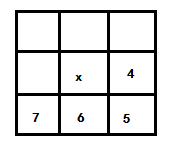完成这两步以后，得到的结果输出即为倒角距离变换的结果。完整的图像倒角距离变换代码实现可以分为如下几步：1.      对像素数组进行初始化，所有背景颜色像素点初始距离为无穷大，前景像素点距	  离为02.      开始倒角距离变换中的第一步，并保存结果3.      基于第一步结果完成倒角距离变换中的第二步4.      根据距离变换结果显示所有不同灰度值，形成图像最终结果显示如下(左边表示原图、右边表示CDT之后结果)完整的二值图像倒角距离变换的源代码如下：package com.gloomyfish.image.transform;

import java.awt.Color;
import java.awt.image.BufferedImage;
import java.util.Arrays;

import com.gloomyfish.filter.study.AbstractBufferedImageOp;

public class CDTFilter extends AbstractBufferedImageOp {
private float[] dis; // nn-distances
private int[] pos; // nn-positions, 32 bit index
private Color bakcgroundColor;

public CDTFilter(Color bgColor)
{
this.bakcgroundColor = bgColor;
}

@Override
public BufferedImage filter(BufferedImage src, BufferedImage dest) {
int width = src.getWidth();
int height = src.getHeight();

if (dest == null)
dest = createCompatibleDestImage(src, null);

int[] inPixels = new int[width * height];
pos = new int[width * height];
dis = new float[width * height];
src.getRGB(0, 0, width, height, inPixels, 0, width);
// 随机生成距离变换点
int index = 0;
Arrays.fill(dis, Float.MAX_VALUE);
int numOfFC = 0;
for (int row = 0; row < height; row++) {
for (int col = 0; col < width; col++) {
index = row * width + col;
if (inPixels[index] != bakcgroundColor.getRGB()) {
dis[index] = 0;
pos[index] = index;
numOfFC++;
}
}
}
final float d1 = 1;
final float d2 = (float) Math.sqrt(d1 * d1 + d1 * d1);
System.out.println(numOfFC);
float nd, nd_tmp;
int i, in, cols, rows, nearestPixel;

// 1 2 3
// 0 i 4
// 7 6 5
// first pass: forward -> L->R, T-B
for (rows = 1; rows < height - 1; rows++) {
for (cols = 1; cols < width - 1; cols++) {
i = rows * width + cols;

nd = dis[i];
nearestPixel = pos[i];
if (nd != 0) { // skip background pixels
in = i;

in += -1; // 0
if ((nd_tmp = d1 + dis[in]) < nd) {
nd = nd_tmp;
nearestPixel = pos[in];
}

in += -width; // 1
if ((nd_tmp = d2 + dis[in]) < nd) {
nd = nd_tmp;
nearestPixel = pos[in];
}

in += +1; // 2
if ((nd_tmp = d1 + dis[in]) < nd) {
nd = nd_tmp;
nearestPixel = pos[in];
}

in += +1; // 3
if ((nd_tmp = d2 + dis[in]) < nd) {
nd = nd_tmp;
nearestPixel = pos[in];
}

dis[i] = nd;
pos[i] = nearestPixel;
}
}
}

// second pass: backwards -> R->L, B-T
// exactly same as first pass, just in the reverse direction
for (rows = height - 2; rows >= 1; rows--) {
for (cols = width - 2; cols >= 1; cols--) {
i = rows * width + cols;

nd = dis[i];
nearestPixel = pos[i];
if (nd != 0) {
in = i;

in += +1; // 4
if ((nd_tmp = d1 + dis[in]) < nd) {
nd = nd_tmp;
nearestPixel = pos[in];
}

in += +width; // 5
if ((nd_tmp = d2 + dis[in]) < nd) {
nd = nd_tmp;
nearestPixel = pos[in];
}

in += -1; // 6
if ((nd_tmp = d1 + dis[in]) < nd) {
nd = nd_tmp;
nearestPixel = pos[in];
}

in += -1; // 7
if ((nd_tmp = d2 + dis[in]) < nd) {
nd = nd_tmp;
nearestPixel = pos[in];
}

dis[i] = nd;
pos[i] = nearestPixel;

}
}
}

for (int row = 0; row < height; row++) {
for (int col = 0; col < width; col++) {
index = row * width + col;
if (Float.MAX_VALUE != dis[index]) {
int gray = clamp((int) (dis[index]));
inPixels[index] = (255 << 24) | (gray << 16) | (gray << 8)
| gray;
}
}
}
setRGB(dest, 0, 0, width, height, inPixels);
return dest;
}

private int clamp(int i) {
return i > 255 ? 255 : (i < 0 ? 0 : i);
}

}转载请注明出处！！
展开全文图像处理 JAVA 算法
• 二值图像距离变换的概念由Rosenfeld和Pfaltz于1966年在论文中提出，目前广泛应用于计算机图形学，目标识别及GIS空间分析等领域，其主要思想是通过表识空间点(目标点与背景点)距离的过程，最终将二值图像转换为...
原文地址
[研究内容]
二值图像距离变换
[正文]
二值图像距离变换的概念由Rosenfeld和Pfaltz于1966年在论文中提出，目前广泛应用于计算机图形学，目标识别及GIS空间分析等领域，其主要思想是通过表识空间点(目标点与背景点)距离的过程，最终将二值图像转换为灰度图像。
距离变换按照距离的类型可以分为欧式距离变换(Eudlidean Distance Transfrom)和非欧式距离变换两种，其中，非欧式距离变换又包括棋盘距离变换(Chessboard Distance Transform)，城市街区距离变换(Cityblock Distance Transform)，倒角距离变换(Chamfer Distance Transform)等；
距离变换的主要过程：
假设一幅二值图像I，包含一个连通区域S，其中有目标集O和背景集B，距离图为D，则距离变换的定义如公式1-(1)：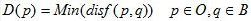1-(1)
具体步骤如下：
1，将图像中的目标像素点分类，分为内部点，外部点和孤立点。
以中心像素的四邻域为例，如果中心像素为目标像素(值为1)且四邻域都为目标像素(值为1)，则该点为内部点。如果该中心像素为目标像素，四邻域为背景像素(值为0)，则该中心点为孤立点，如下图Fig.1所示。除了内部点和孤立点之外的目标区域点为边界点。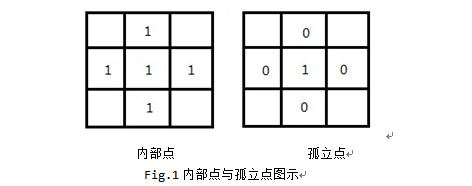2，计算图像中所有的内部点和非内部点，点集分别为S1，S2。
3，对于S1中的每一个内部点(x,y)，使用距离公式disf()计算骑在S2中的最小距离，这些最小距离构成集合S3。
4，计算S3中的最大最小值Max,Min。
5，对于每一个内部点，转换后的灰度值G计算如下公式1-(2)所示：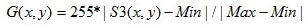1-(2)
其中，S3(x,y)表示S1中的点(x,y)在S2中的最短距离
6，对于孤立点保持不变。
在以上距离变换的过程中，距离函数disf()的选取如果是欧式距离，则该距离变换称为欧式距离变换，依次类推。对于距离的求取，目前主要的距离公式如下：
欧式距离：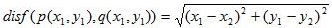1-(3)
棋盘距离：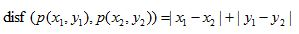1-(4)
城市街区距离：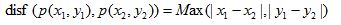1-(5)
对于欧式距离变换，由于其结果准确，而计算相比非欧式距离变换较为复杂，因此，出现了较多的快速欧式距离变换算法，这里笔者介绍一种基于3*3模板的快速欧式距离变换算法(文献2)，具体过程如下：
1，按照从上到下，从左到右的顺序，使用模板如图Fig.2，依次循环遍历图像I，此过程称为前向循环。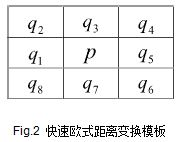对于p对应的像素(x,y)，我们计算五个距离：d0,d1,d2,d3,d4：
d0=p(x,y)
d1=p(x-1,y)+disf((x-1,y),(x,y))
d2=p(x-1,y-1)+disf((x-1,y-1),(x,y))
d3=p(x,y-1)+disf((x,y-1),(x,y))
d4=p(x+1,y-1)+disf((x-1,y-1),(x,y))
则p(x,y)变换后的像素值为：
p(x,y)=Min(d0,d1,d2,d3,d4);
使用上述算法得到图像I'。
2，按照从下到上，从右到左的顺序，使用Fig.2所示模板依次循环遍历图像I’，此过程称为后向循环。
对于p对应的像素(x,y)，我们计算五个距离：d0,d5,d6,d7,d8：
d0=p(x,y)
d5=p(x+1,y)+disf((x+1,y),(x,y))
d6=p(x+1,y+1)+disf((x+1,y+1),(x,y))
d7=p(x,y+1)+disf((x,y+1),(x,y))
d8=p(x-1,y+1)+disf((x-1,y+1),(x,y))
则p(x,y)后向变换后的像素值为：
p(x,y)=Min(d0,d5,d6,d7,d8);
使用上述算法得到的图像即为距离变换得到的灰度图像。
以上过程即文献2所示快速欧式距离变换算法。如果我们需要非欧氏距离变换的快速算法，只需要修改文献2算法中的欧式距离公式disf()为非欧式距离公式，如棋盘距离，城市街区距离等，过程依次类推。
对于欧式距离变换算法，相关学者研究了速度更快的倒角距离变换算法，来近似欧式距离变换的效果。具体过程如下：
1，使用前向模板如图Fig.3中左边3*3模板，对图像从上到下，从左到右进行扫描，模板中心0点对应的像素值如果为0则跳过，如果为1则计算模板中每个元素与其对应的像素值的和，分别为Sum1,Sum2,Sum3,Sum4，Sum5，而中心像素值为这五个和值中的最小值。
2，使用后向模板如图Fig.3中右边的3*3模板，对图像从下到上，从右到左进行扫描，方法同上。
3，一般我们使用的倒角距离变换模板为3*3和5*5，分别如下图所示：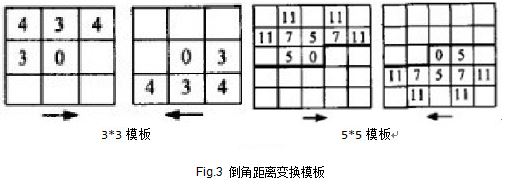[实验结果]
实验采用512*512大小的图像进行测试，测试PC为64位，Intel(R) Core(TM) i5-3230 CPU, 2.6GHz, 4G RAM，运行环境为VS2008，C#。
实验结果如下：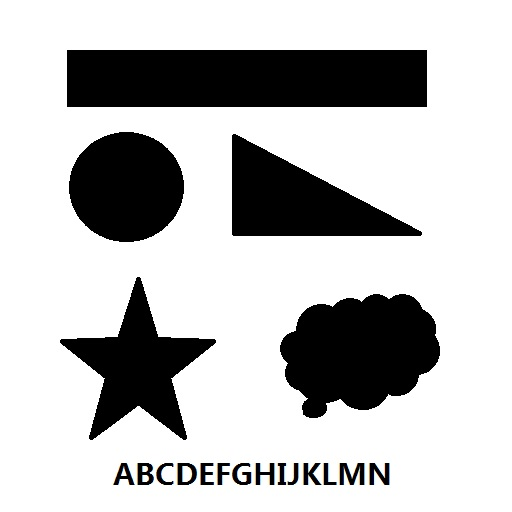(a)原图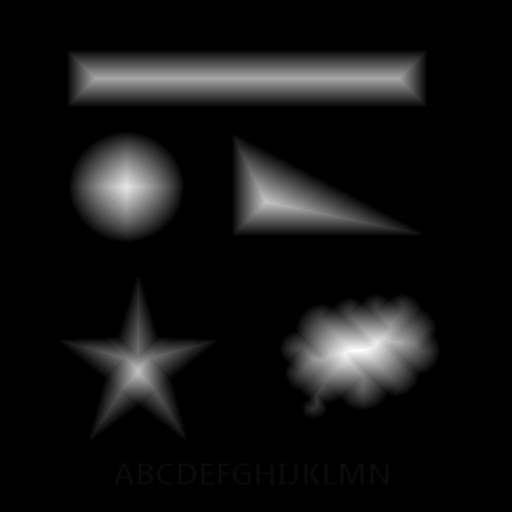(b)Euclidean Distance Transfrom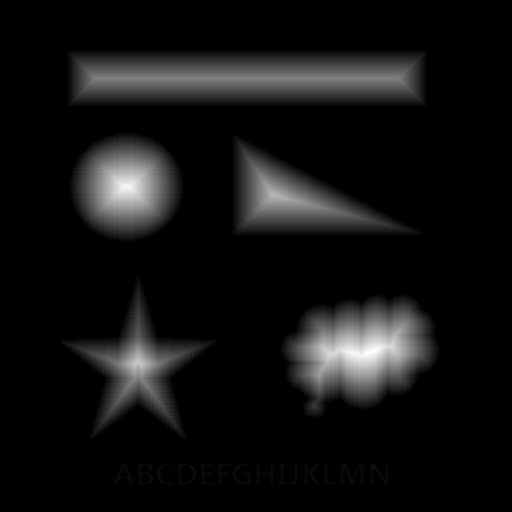(c) Cityblock  Distance Transfrom(d) Chessboard Distance Transform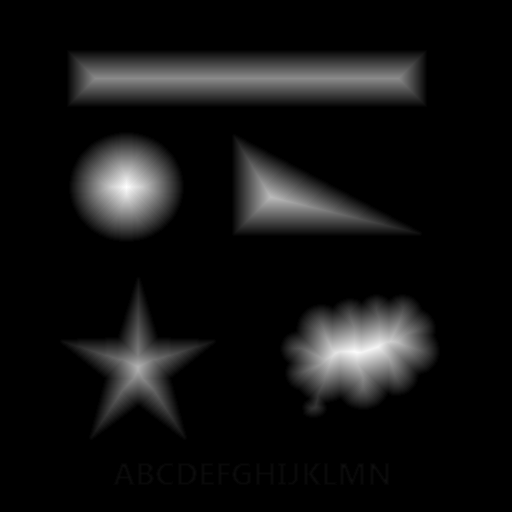(e) Chamfer Distance Transform
对于以上欧式距离变换与非欧式距离变换，我们做了时间分析，结果如下：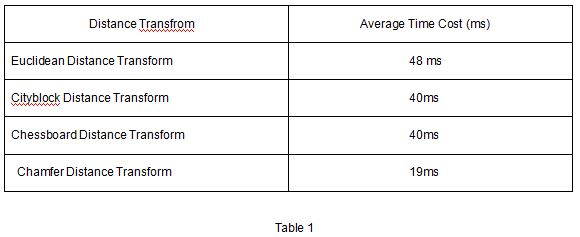对于Table 1的数据，是通过计算50张512*512大小的图像得到的平均结果，代码未曾优化，距离变换结果均做了均衡化处理，对于不同配置，不同程序语言可能存在一定差异，总体而言，基于3*3模板的倒角距离变换速度最快，大概是欧氏距离快速算法的一半。
[参考文献]
 Rosenfeld A,PfaltzJ.L, Sequential operations in digital pic ture processing. Journal of ACM,1966, 13(4):471-494.
 Frank Y.Shih,Yi-Ta Wu, Fast Euclidean distance transformation in two scans using a 3*3 neighborhood. Journal of Computer Vision and Image Understanding 2004,195–205.
附录
本人使用C#编写的代码如下：

[csharp] view
plain copy/// <summary>
/// Distance transform for binary image.
/// </summary>
/// <param name="src">The source image.</param>
/// <param name="distanceMode">One parameter to choose the mode of distance transform from 1 to 3, 1 means Euclidean Distance, 2 means CityBlock Distance, 3 means ChessBoard Distance.</param>
/// <returns>The result image.</returns>
public Bitmap DistanceTransformer(Bitmap src, int distanceMode)
{
int w = src.Width;
int h = src.Height;
double p0 = 0, p1 = 0, p2 = 0, p3 = 0, p4 = 0, p5 = 0, p6 = 0, p7 = 0, p8 = 0, min = 0;
int mMax = 0, mMin = 255;
System.Drawing.Imaging.BitmapData srcData = src.LockBits(new Rectangle(0, 0, w, h), System.Drawing.Imaging.ImageLockMode.ReadOnly, System.Drawing.Imaging.PixelFormat.Format24bppRgb);
unsafe
{
byte* p = (byte*)srcData.Scan0.ToPointer();
int stride = srcData.Stride;
byte* pTemp;
for (int y = 0; y < h; y++)
{
for (int x = 0; x < w; x++)
{
if (x > 0 && x < w - 1 && y > 0 && y < h - 1)
{
p0 = (p + x * 3 + y * stride);
if (p0 != 0)
{
pTemp = p + (x - 1) * 3 + (y - 1) * stride;
p2 = pTemp + GetDistance(x, y, x - 1, y - 1, distanceMode);
pTemp = p + x * 3 + (y - 1) * stride;
p3 = pTemp + GetDistance(x, y, x, y - 1, distanceMode);
pTemp = p + (x + 1) * 3 + (y - 1) * stride;
p4 = pTemp + GetDistance(x, y, x + 1, y - 1, distanceMode);
pTemp = p + (x - 1) * 3 + y * stride;
p1 = pTemp + GetDistance(x, y, x - 1, y, distanceMode);
min = GetMin(p0, p1, p2, p3, p4);
pTemp = p + x * 3 + y * stride;
pTemp = (byte)Math.Min(min, 255);
pTemp = (byte)Math.Min(min, 255);
pTemp = (byte)Math.Min(min, 255);
}
}
else
{
pTemp = p + x * 3 + y * stride;
pTemp = 0;
pTemp = 0;
pTemp = 0;
}

}

}
for (int y = h - 1; y > 0; y--)
{
for (int x = w - 1; x > 0; x--)
{
if (x > 0 && x < w - 1 && y > 0 && y < h - 1)
{
p0 = (p + x * 3 + y * stride);
if (p0 != 0)
{
pTemp = p + (x + 1) * 3 + y * stride;
p5 = pTemp + GetDistance(x, y, x + 1, y, distanceMode);
pTemp = p + (x + 1) * 3 + (y + 1) * stride;
p6 = pTemp + GetDistance(x, y, x + 1, y + 1, distanceMode);
pTemp = p + x * 3 + (y + 1) * stride;
p7 = pTemp + GetDistance(x, y, x, y + 1, distanceMode);
pTemp = p + (x - 1) * 3 + (y + 1) * stride;
p8 = pTemp + GetDistance(x, y, x - 1, y + 1, distanceMode);
min = GetMin(p0, p5, p6, p7, p8);
pTemp = p + x * 3 + y * stride;
pTemp = (byte)Math.Min(min, 255);
pTemp = (byte)Math.Min(min, 255);
pTemp = (byte)Math.Min(min, 255);
mMax = (int)Math.Max(min, mMax);
}
}
else
{
pTemp = p + x * 3 + y * stride;
pTemp = 0;
pTemp = 0;
pTemp = 0;
}

}

}
mMin = 0;
for (int y = 0; y < h; y++)
{
for (int x = 0; x < w; x++)
{
pTemp = p + x * 3 + y * stride;
if (pTemp != 0)
{
int temp = pTemp;
pTemp = (byte)((temp - mMin) * 255 / (mMax - mMin));
pTemp = (byte)((temp - mMin) * 255 / (mMax - mMin));
pTemp = (byte)((temp - mMin) * 255 / (mMax - mMin));
}
}

}
src.UnlockBits(srcData);
return src;
}
}
/// <summary>
/// Chamfer distance transform(using two 3*3 windows:forward window434 300 000;backward window 000 003 434).
/// </summary>
/// <param name="src">The source image.</param>
/// <returns>The result image.</returns>
public Bitmap ChamferDistancetransfrom(Bitmap src)
{
int w = src.Width;
int h = src.Height;
double p0 = 0, p1 = 0, p2 = 0, p3 = 0, p4 = 0, p5 = 0, p6 = 0, p7 = 0, p8 = 0, min = 0;
int mMax = 0, mMin = 255;
System.Drawing.Imaging.BitmapData srcData = src.LockBits(new Rectangle(0, 0, w, h), System.Drawing.Imaging.ImageLockMode.ReadOnly, System.Drawing.Imaging.PixelFormat.Format24bppRgb);
unsafe
{
byte* p = (byte*)srcData.Scan0.ToPointer();
int stride = srcData.Stride;
byte* pTemp;
for (int y = 0; y < h; y++)
{
for (int x = 0; x < w; x++)
{
if (x > 0 && x < w - 1 && y > 0 && y < h - 1)
{
p0 = (p + x * 3 + y * stride);
if (p0 != 0)
{
pTemp = p + (x - 1) * 3 + (y - 1) * stride;
p2 = pTemp + 4;
pTemp = p + x * 3 + (y - 1) * stride;
p3 = pTemp + 3;
pTemp = p + (x + 1) * 3 + (y - 1) * stride;
p4 = pTemp + 4;
pTemp = p + (x - 1) * 3 + y * stride;
p1 = pTemp + 3;
min = GetMin(p0, p1, p2, p3, p4);
pTemp = p + x * 3 + y * stride;
pTemp = (byte)Math.Min(min, 255);
pTemp = (byte)Math.Min(min, 255);
pTemp = (byte)Math.Min(min, 255);
}
}
else
{
pTemp = p + x * 3 + y * stride;
pTemp = 0;
pTemp = 0;
pTemp = 0;
}

}

}
for (int y = h - 1; y > 0; y--)
{
for (int x = w - 1; x > 0; x--)
{
if (x > 0 && x < w - 1 && y > 0 && y < h - 1)
{
p0 = (p + x * 3 + y * stride);
if (p0 != 0)
{
pTemp = p + (x + 1) * 3 + y * stride;
p5 = pTemp + 3;
pTemp = p + (x + 1) * 3 + (y + 1) * stride;
p6 = pTemp + 4;
pTemp = p + x * 3 + (y + 1) * stride;
p7 = pTemp + 3;
pTemp = p + (x - 1) * 3 + (y + 1) * stride;
p8 = pTemp + 4;
min = GetMin(p0, p5, p6, p7, p8);
pTemp = p + x * 3 + y * stride;
pTemp = (byte)Math.Min(min, 255);
pTemp = (byte)Math.Min(min, 255);
pTemp = (byte)Math.Min(min, 255);
mMax = (int)Math.Max(min, mMax);
}
}
else
{
pTemp = p + x * 3 + y * stride;
pTemp = 0;
pTemp = 0;
pTemp = 0;
}

}

}
mMin = 0;
for (int y = 0; y < h; y++)
{
for (int x = 0; x < w; x++)
{
pTemp = p + x * 3 + y * stride;
if (pTemp != 0)
{
int temp = pTemp;
pTemp = (byte)((temp - mMin) * 255 / (mMax - mMin));
pTemp = (byte)((temp - mMin) * 255 / (mMax - mMin));
pTemp = (byte)((temp - mMin) * 255 / (mMax - mMin));
}
}

}
src.UnlockBits(srcData);
return src;
}
}
private double GetDistance(int x, int y, int dx, int dy, int mode)
{
double result = 0;
switch (mode)
{
case 1:
result = EuclideanDistance(x, y, dx, dy);
break;
case 2:
result = CityblockDistance(x, y, dx, dy);
break;
case 3:
result = ChessboardDistance(x, y, dx, dy);
break;
}
return result;
}
private double EuclideanDistance(int x, int y, int dx, int dy)
{
return Math.Sqrt((x - dx) * (x - dx) + (y - dy) * (y - dy));
}
private double CityblockDistance(int x, int y, int dx, int dy)
{
return Math.Abs(x - dx) + Math.Abs(y - dy);
}
private double ChessboardDistance(int x, int y, int dx, int dy)
{
return Math.Max(Math.Abs(x - dx), Math.Abs(y - dy));
}
private double GetMin(double a, double b, double c, double d, double e)
{
return Math.Min(Math.Min(Math.Min(a, b), Math.Min(c, d)), e);
}

最后，分享一个专业的图像处理网站（微像素），里面有很多源代码下载：

http://www.zealpixel.com/portal.php

展开全文• 倒角距离变换(Chamfer Distance Transform) 图a,b为距离变换模板，设其值分别为a[1-5],b[5-9]。 图c为待处理的二维二值化图像，其中1代表目标区域，0代表背景区域。设其值为c[m][n]。 目标是求出目标区域中的每一个...倒角距离变换
• 主要使用傅里叶变换，将空间域的图像转换到频域内，在频域内进行数字图像处理。这部分的内容及其重要，频域内的处理可以解决空间域内无法完成的图像增强。本文首先从数学角度，对图像的频域内的性质进行分析，然后在...图像处理 MATLAB 低通滤波器
• ## 全景视频拼接关键技术

万次阅读 多人点赞 2013-10-17 17:50:29
图像拼接(Image Stitching)是一种利用实景图像组成全景空间的技术，它将多幅图像拼接成一幅大尺度图像或360度全景图，图像拼接技术涉及到计算机视觉、计算机图形学、数字图像处理以及一些数学工具等技术。...态势感知
• 一、距离定义 （1）欧式距离  ，实质上是矩形对线距离 （2）city block ，实质上是从一个点到另外一个点的步数，并不能走斜线 （3）chessboard ，实质上是凑成一个正方形的对线 （4）邻接性  4-连接没有...
• 1、象素间各种距离的定义及计算 我们知道，构成一幅数字图像最基本的元素是一个一个的像素点，也就是像素。理解像素间的一些基本关系是我们以后进行图形图形处理的基础和关键。如相邻像素（像素的邻域），像素的...
• 介绍了无人机图像拼接一些流程和常用方法，可以通过运动估计来实现图像的拼接算法 图像拼接
• ## 图像拼接

万次阅读 2015-11-24 22:07:17
(1) 图像预处理：对原始图像进行直方图匹配、平滑滤波、增强变换等数字图像 处理的基本操作，为图像拼接的下一步作好准备。 (2) 图像配准：图像配准是整个图像拼接流程的核心，配准的精度决定了图像的拼接质量。...
• 本文主要讲述基于VC++6.0 MFC图像处理的应用知识，主要结合自己大三所学课程《数字图像处理》及课件进行讲解，主要通过MFC单文档视图实现显示BMP图片空间几何变换，包括图像平移、图形旋转、图像反转倒置镜像和图像...图像处理 几何变换
•  在之前的文中，我们已经进行过一些基本的图像处理。比如，使用低通滤波可以将图像模糊，也有些许降噪的作用。这些都是在空间域内进行的滤波处理，这个处理主要是依靠卷积来进行计算的。首先，从连续的一维卷积入手...图像处理 傅里叶变换 低通滤波器
• ## Keras ImageDataGenerator参数

万次阅读 多人点赞 2018-02-03 14:17:31
from keras.preprocessing.image import ImageDataGenerator keras.preprocessing.image.ImageDataGenerator(featurewise_center=False, samplewise_center=False, featurewise_std_normalization = False,
• ## SFM原理简介

万次阅读 多人点赞 2019-10-24 14:32:24
Structure From Motion SFM简介 ...通过相机的移动来确定目标的空间和几何关系，是三维重建的一种常见方法。它与Kinect这种3D摄像头最大的不同在于，它只需要普通的RGB摄像头即可，因此成本更低廉，且受环境...
• [OpenGL ES 03]3D变换：模型，视图，投影与Viewport 罗朝辉 (http://blog.csdn.net/kesalin) 本文遵循“署名-非商业用途-保持一致”创作公用协议 系列文章： [OpenGL ES 01]OpenGL ES初体验 [OpenGL ES 02]...3D
• 笔者在这几天看了一些关于图像特征化的内容，发现图像相似性搜索领域，一般先图像特征量化，然后进行相应的匹配。 1、方向一：描述符。先抽取图像局部特征符，根据一些匹配算法进行匹对，较多适合在图像比对，...相似性 图像 哈希值 检索
• ## 三维重建-opencv实现sfm

万次阅读 多人点赞 2016-07-03 22:23:08
注意：本文中的代码必须使用OpenCV3.0或以上版本进行编译，因为很多函数是3.0以后才加入的。 目录： ...SfM介绍小孔相机模型坐标系内参矩阵外参矩阵相机的标定 ...SfM的全称为Structure from Motion，即通过相机的...
• 在网上看到python做图像识别的相关文章后，真心感觉python的功能实在太强大，因此将这些文章总结一下，建立一下自己的知识体系。 当然了，图像识别这个话题作为计算机科学的一个分支，不可能就在本文简单几句就说清...python 识别 相似图片 图片搜索
• ## Python+Opencv进行识别相似图片

万次阅读 多人点赞 2016-04-29 10:22:44
在网上看到python做图像识别的相关文章后，真心感觉python的功能实在太强大，因此将这些文章总结一下，建立一下自己的知识体系。 当然了，图像识别这个话题作为计算机科学的一个分支，不可能就在本文简单几句就说清...
...